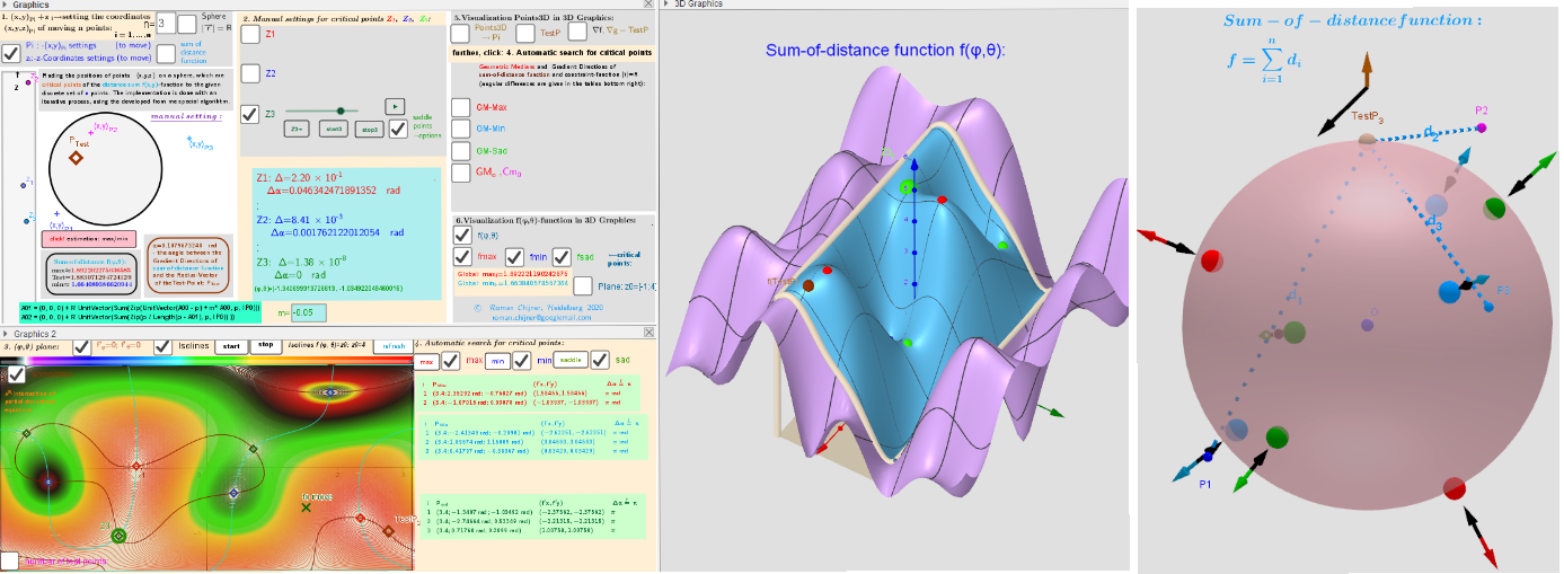# Example 1 of Applet in which 3 moving points in three-dimensional space "induce" 8 geometric medians on a sphere.

Let Pi=(xi,yi,zi) n moving points in ℝ³ (lP:={P1,P2,...,Pn}). I want to find  the points P=(x,y,z) on the surface of the sphere -S (radius R) that are critical (relative min/max or saddle points at (x,y,z)) of a function f(x,y,z) is the sum of the distances from P to the all points from lP. Critical points can be found using Lagrange multipliersas finding the Extreme values of the function f(x,y,z) subject to a constraining equation g(x,y,z):=x2+y2+z2-R2=0. There is a system of equations: ∇f(x,y,z)= λ∇g(x,y,z). A local optimum occurs when ∇f(x,y,z) and ∇g(x,y,z) are parallel, and so ∇f is some multiple of ∇g. If we substitute the variable z=R cos(θ), then we will have a two-variable function f(φ,θ) over a rectangular region: - π ≤φ≤ π; -π/2≤θ≤π/2. The solution of the system of equations can be found as the intersection points of the corresponding implicit functions. The point P=(x,y,z)∈ ℝ³ that minimize a function of the sum of distances from this point to all points lP is called their Fermat's point or the Geometric Median. In our case P=(x,y,z)∈S! There are no explicit Geometric Medians formulas, in contrast to Geometric Centers explicit formulas. The solution of the system of equations can be found use iterative procedures. Here you can visually observe and explore these solutions in different positions of 3 moving points from the lP set﻿. Description is in https://www.geogebra.org/m/y8dnkeuu.- Intersection Implicit Curves f'φ(φ, θ)=0; f'θ(φ,θ)=0 over a rectangular region φ∈[-π,π], θ∈[-0.5π,0.5π] - Critical points on the surface of distance sum function f(φ,θ) over a rectangular region φ∈[-π,π], θ∈[-0.5π,0.5π] - Distribution of points Pi and their local maxima/minima and saddle -critical points of distance sum function f(φ,θ) on a sphere + test Point. Vectors ∇f and ∇g at these points.